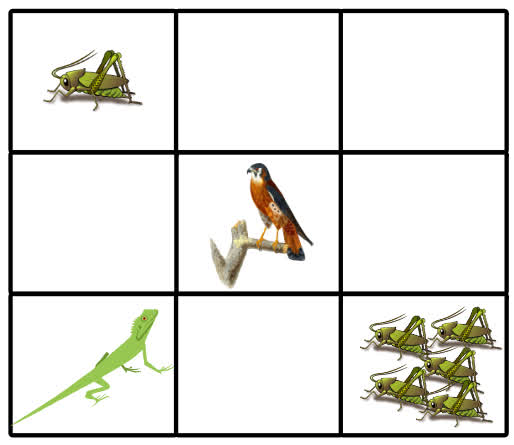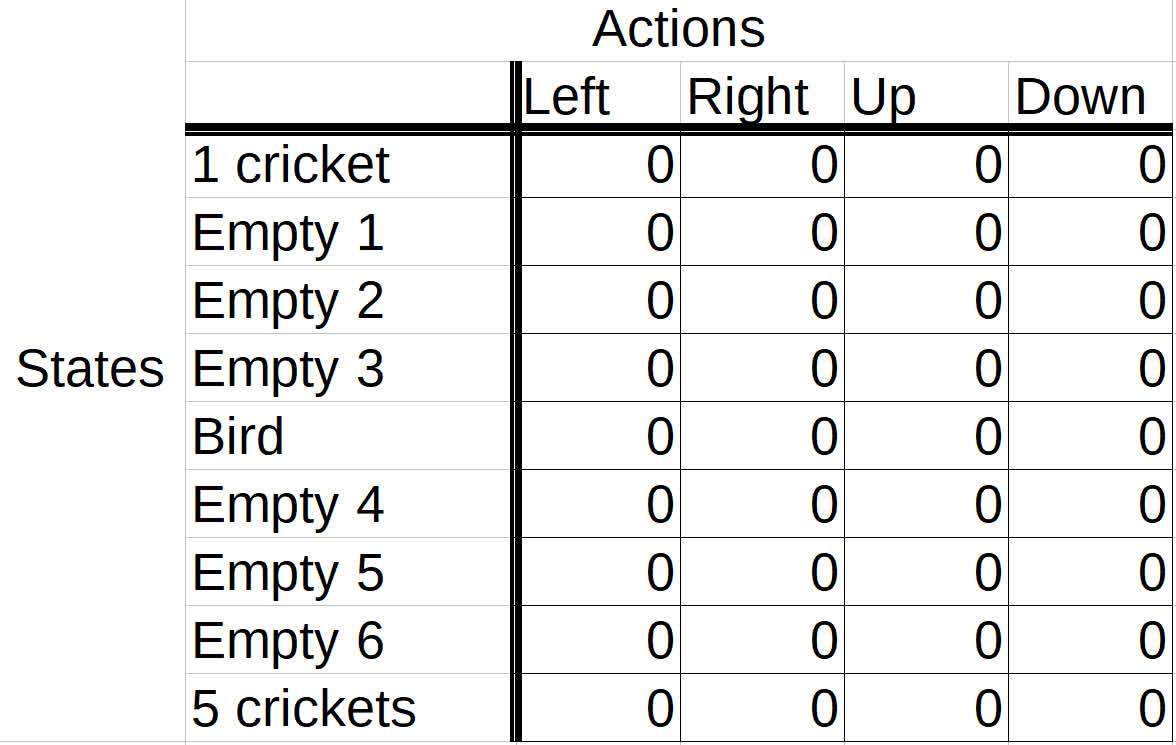# Reinforcement Learning - Goal Oriented Intelligence

Deep Learning Course 4 of 5 - Level: Advanced

## Q-Learning Explained - A Reinforcement Learning Technique

### video

expand_more chevron_left

### text

expand_more chevron_left

### Introduction to Q-learning and Q-tables

What's up, guys? In this post, we'll be introducing the idea of Q-learning, which is a reinforcement learning technique used for learning the optimal policy in a Markov Decision Process. We'll illustrate how this technique works by introducing a game where a reinforcement learning agent tries to maximize points. So, let's get to it!Last time, we left off talking about the fact that once we have our optimal Q-function $$q_*$$ we can determine the optimal policy by applying a reinforcement learning algorithm to find the action that maximizes $$q_*$$ for each state.

### Q-learning objective

Q-learning is the first technique we'll discuss that can solve for the optimal policy in an MDP.

The objective of Q-learning is to find a policy that is optimal in the sense that the expected value of the total reward over all successive steps is the maximum achievable. So, in other words, the goal of Q-learning is to find the optimal policy by learning the optimal Q-values for each state-action pair.

Let's now explore how Q-learning works!

### Q-learning with value iteration

First, as a quick reminder, remember that the Q-function for a given policy accepts a state and an action and returns the expected return from taking the given action in the given state and following the given policy thereafter.

Also, remember this Bellman optimality equation for $$q_*$$ we discussed last time? \begin{eqnarray*} q_{\ast }\left( s,a\right) &=&E\left[ R_{t+1}+\gamma \max_{a^{\prime }}q_{\ast }\left( s^\prime,a^{\prime }\right)\right] \end{eqnarray*} Go take a peak at the explanation we gave previously for this equation if you're a bit rusty on how to interpret this. It will become useful in a moment.

### Value iteration

The Q-learning algorithm iteratively updates the Q-values for each state-action pair using the Bellman equation until the Q-function converges to the optimal Q-function, $$q_*$$. This approach is called value iteration. To see exactly how this happens, let's set up an example, appropriately called The Lizard Game.

### The set up

Suppose we have the following environment shown below. The agent in our environment is the lizard. The lizard wants to eat as many crickets as possible in the least amount of time without stumbling across a bird, which will, itself, eat the lizard.The lizard can move left, right, up, or down in this environment. These are the actions. The states are determined by the individual tiles and where the lizard is on the board at any given time.

If the lizard lands on a tile that has one cricket, the reward is plus one point. Landing on an empty tile is minus one point. A tile with five crickets is plus ten points and will end the episode. A tile with a bird is minus ten points and will also end the episode.

State Reward
One cricket +1
Empty - 1
Five crickets +10 Game over
Bird - 10 Game over

Now, at the start of the game, the lizard has no idea how good any given action is from any given state. It's not aware of anything besides the current state of the environment. In other words, it doesn't know from the start whether navigating left, right, up, or down will result in a positive reward or negative reward.

Therefore, the Q-values for each state-action pair will all be initialized to zero since the lizard knows nothing about the environment at the start. Throughout the game, though, the Q-values will be iteratively updated using value iteration.

### Storing Q-values in a Q-table

We'll be making use of a table, called a Q-table, to store the Q-values for each state-action pair. The horizontal axis of the table represents the actions, and the vertical axis represents the states. So, the dimensions of the table are the number of actions by the number of states.As just mentioned, since the lizard knows nothing about the environment or the expected rewards for any state-action pair, all the Q-values in the table are first initialized to zero. Over time, though, as the lizard plays several episodes of the game, the Q-values produced for the state-action pairs that the lizard experiences will be used to update the Q-values stored in the Q-table.

As the Q-table becomes updated, in later moves and later episodes, the lizard can look in the Q-table and base its next action on the highest Q-value for the current state. This will make more sense once we actually start playing the game and updating the table.

#### Episodes

Now, we'll set some standard number of episodes that we want the lizard to play. Let's say we want the lizard to play five episodes. It is during these episodes that the learning process will take place.

In each episode, the lizard starts out by choosing an action from the starting state based on the current Q-values in the table. The lizard chooses the action based on which action has the highest Q-value in the Q-table for the current state.

But, wait... That's kind of weird for the first actions in the first episode, right? Because all the Q-values are set zero at the start, so there's no way for the lizard to differentiate between them to discover which one is considered better. So, what action does it start with?

To answer this question, we'll introduce the trade-off between exploration and exploitation. This will help us understand not just how an agent takes its first actions, but how exactly it chooses actions in general.

### Exploration vs. exploitation

Exploration is the act of exploring the environment to find out information about it. Exploitation is the act of exploiting the information that is already known about the environment in order to maximize the return.The goal of an agent is to maximize the expected return, so you might think that we want our agent to use exploitation all the time and not worry about doing any exploration. This strategy, however, isn't quite right.

Think of our game. If our lizard got to the single cricket before it got to the group of five crickets, then only making use of exploitation, going forward the lizard would just learn to exploit the information it knows about the location of the single cricket to get single incremental points infinitely. It would then also be losing single points infinitely just to back out of the tile before it can come back in to get the cricket again.

If the lizard was able to explore the environment, however, it would have the opportunity to find the group of five crickets that would immediately win the game. If the lizard only explored the environment with no exploitation, however, then it would miss out on making use of known information that could help to maximize the return.

Given this, we need a balance of both exploitation and exploration. So how do we implement this?

### Wrapping up

To get this balance between exploitation and exploration, we use what is called an epsilon greedy strategy, and that is actually where we'll be picking up in the next post! There, we'll learn all about how an agent, the lizard in our case, chooses to either explore or exploit the environment.Thanks for contributing to collective intelligence, and I'll see ya in the next one!

### quiz

expand_more chevron_leftDEEPLIZARD Message notifications

### resources

expand_more chevron_left

expand_more chevron_leftDEEPLIZARD Message notifications